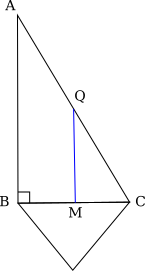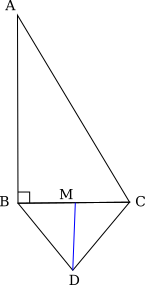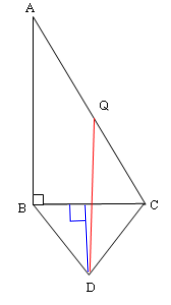#### You may also like### Doodles

Draw a 'doodle' - a closed intersecting curve drawn without taking pencil from paper. What can you prove about the intersections?### Russian Cubes

I want some cubes painted with three blue faces and three red faces. How many different cubes can be painted like that?### Picture Story

Can you see how this picture illustrates the formula for the sum of the first six cube numbers?

# Parallel Universe

##### Age 14 to 16 Challenge Level:

A simple way to solve this is by considering M, the midpoint of BD:The line joining M and Q (left) creates triangle QMC, which is similar to triangle ABC. So QMC is a right-angled triangle, with QM perpendicular to BC.

The line joining M and D (right) is a line of symmetry of triangle BCD. So MD is perpendicular to BC.

Since QM and MD are both perpendicular to BC, QMD is a straight line. So M lies on QD, and QD is perpendicular to BC.

But AB is also perpendicular to BC, so QD is parallel to AB.

Emily from Wimbledon High School solved this by using vectors to find the cartesian co-ordinates of the points.

Let $B$ be the origin. Then align the triangles so $A$ is vertically above $B$, so the co-ordinates of $A$ are $(0,a)$.By the right angle $C$ is horizontally across from $B$, so $C$ is $(c,0)$. Then $Q$ is $\left(\frac{c}{2},\frac{a}{2}\right)$.

By some basic trigonometry we can find the coordinates of $D$, as $BCD$ is an equilateral triangle we know all its angles are $60^\circ$ and all its sides are equal. By considering the perpendicular from $BC$ to $D$, we find the horizontal component of $D$ is $d\times \cos{60}$ and the length $BD=d$ is equal to $BC=c$ so $d\times \cos{60}=\frac{c}{2}$. The vertical component is $d\times \sin{60}=c\frac{3^{1/2}}{2}$.

But the vertical component does not matter, the point is that $D$ and $Q$ have the same horizontal component, so a straight line between them will cross through $BC$ and will be vertical, and hence parallel to $BA$